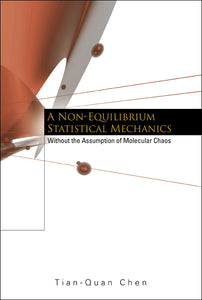# Non-equilibrium Statistical Mechanics, A: Without The Assumption Of Molecular Chaos

Tian-quan Chen

Format: Print Book

ISBN: 9789812383785

• SGD 228.98
Unit price per
Tax included.

This book presents the construction of an asymptotic technique for solving the Liouville equation, which is to some degree an analogue of the Enskog-Chapman technique for solving the Boltzmann equation. Because the assumption of molecular chaos has been given up at the outset, the macroscopic variables at a point, defined as arithmetic means of the corresponding microscopic variables inside a small neighborhood of the point, are random in general. They are the best candidates for the macroscopic variables for turbulent flows. The outcome of the asymptotic technique for the Liouville equation reveals some new terms showing the intricate interactions between the velocities and the internal energies of the turbulent fluid flows, which have been lost in the classical theory of BBGKY hierarchy.

Format: Hardcover
No of Pages: 436
Imprint: World Scientific
Publication date: 20030709